Mathematics

# One to one vs Onto vs Bijective

4k views

## Table of Contents

 1 Introduction 2 Function vs not a Function 3 One to One Vs Onto 4 Both One to One and Onto 5 Into vs Onto 6 Summary 7 FAQs

1 October 2020

Read time: 3 minutes

## Introduction

Let’s say you are thirsty and want to drink apple juice. You get your juicer machine out and prepare to make the apple juice. All you have to do is put an apple into the juicer, and apple juice will come out of the juicer outlet.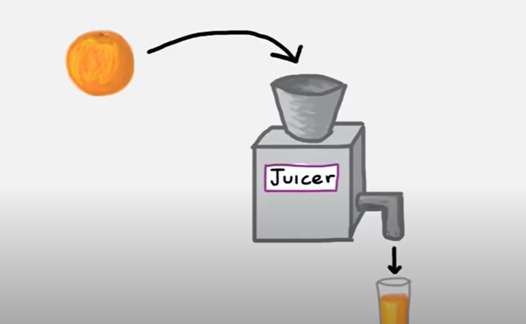Here the input will be the apple, and the output will be the apple juice. Notice that if you put grapes in the juicer, you couldn’t expect to get apple juice out of the juicer; you will get grape juice. Similarly, if you place an orange in the juicer, you will get orange juice.

Your juicer here is acting like a function y = f(x) where the x is the fruit you put in the juicer, f is the juicer, and y is the output juice. This is the simple concept of the function, you put something in, and you get something out.

In this blog, we will cover various aspects of function like how to know if a relation is a function, the difference between multiple types of functions like one to one vs onto, etc.

## Function vs not a Function

Now, let us talk about how to recognize what is a function or Function vs not a function. Function vs not a function is an important topic, and it strengthens your fundamental understanding of the topic.

A function takes an input and produces a unique output while, on the other hand, a non-function can produce different outputs for the same input. For example, $$y^2=x,$$ if you put x = 9, you will get two answers for y, which are 3 and -3. So $$y^2=x,$$ is not a function.

Another example, $$y=x,$$ always has a unique y corresponding to each x. So, it can be called a function. There is a test for checking if a particular relationship is a function or not. It is called the vertical test.

In the vertical test, we plot an x-y graph of the function, and then we move a vertical line from left to right( or vice-versa) on the graph. If the vertical line cuts the graph at more than one point at any instant, the given relationship is not a function. (refer above image). This way you can decide function vs not a function for any relation.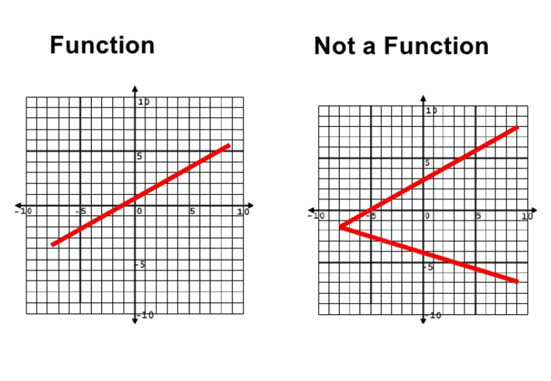Also, it can be explained with the mapping of x and y. If there is exactly one line originating from each x, then it is a function. If multiple lines are originating from one x, it is not a function.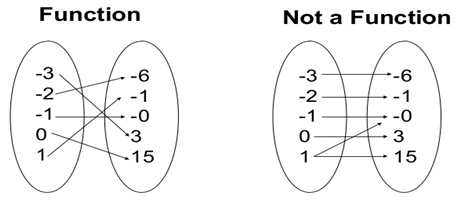## One to One Vs Onto

One to One vs. Onto function is a simple concept, but sometimes it gets confusing for students. One to One is also an essential prerequisite for learning about inverse functions. It is also known as Injective function.

For understanding One to One vs onto functions, let’s go back to the juicer example. We cannot get orange juice from an apple as input fruit or grape juice as output after putting in the apple in the juicer.

Thus, we can say that there is one unique output for each fruit input that cannot be obtained by any other fruit as input. One to One functions behaves very similarly to these.

For a given y, there is only one x that can be paired with the given y. If any two or more elements of set x are connected with a single element of the set y, then we call this function as Many to one function. In the below image, you can see that each point on the left side(x) is connected to exactly one element on the right side(y). Hence, it is One to One function.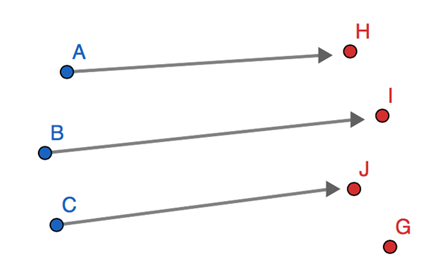For example, the function is not a one to one function as y is the same for $$x=1,$$ and $$x=-1$$ . On the other hand, $$y=x^3$$ is a One to One function.

To know if a particular function is One to One or not, you can perform the horizontal line test. It is similar to the vertical line test. On an x-y graph of the given function, move the horizontal line from top to bottom; if it cuts more than one point on the graph at any instance, the function is not One to One. It will be called a Many to One function instead. This can also decipher one to one vs onto to some level.

For a function, $$y=f(x)$$ , the range of values x can take is called the domain of function f, and the range of values taken by y is called co-domain of the function f.

A function can be called Onto function when there is a mapping to an element in the domain for every element in the co-domain. It merely means that every value in the output set is connected to the input; no output values remain unconnected. Onto functions are also referred to as Surjective functions. In the below image, you can see that each value on the right side(y) is connected to the left side(x).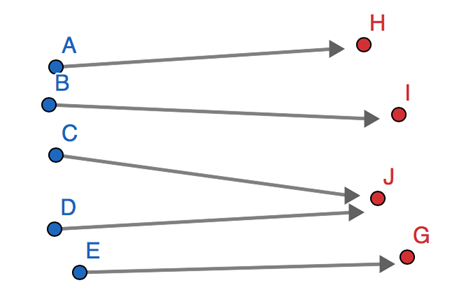Hopefully, this clears the difference of onto vs one to one.

## Both One to One and Onto

In the previous section, we discussed One to One vs Onto functions. Now, what happens when a function is both One to One and Onto?

A function that is both One to One and Onto is called Bijective function. Each value of the output set is connected to the input set, and each output value is connected to only one input value.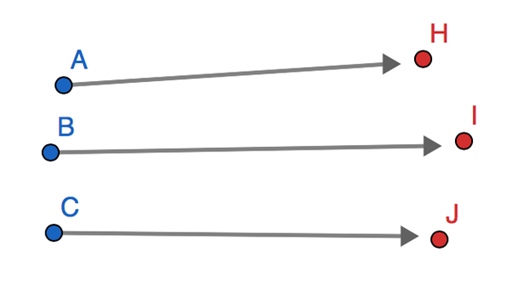In the above image, you can see that each element on left is connected exactly once to the right side, hence one to one, and each element on the right side is connected to the left side, hence Onto. As it is both One to One and Onto, it is said to be Bijective.

For example, the function $$y=x$$ is also both One to One and Onto; hence it is bijective.Bijective functions are special classes of functions; they are said to have an inverse.

## Into vs Onto Function

Let us now discuss the difference between Into vs Onto function. For Onto functions, each element of the output set y should be connected to the input set. On the flip side, for Into functions, there should be at least one element in the output set y that is not connected to the input set.

## Summary

This blog covered different types of functions, functions vs non-function, One to One vs Onto, Into vs Onto, etc. with some simple examples. These concepts are quite fundamental to mathematics and should not be overlooked. They are the base for some complex mathematical topics. We highly encourage you to take this as a starting point and read more about these topics.

## What is the difference between Surjective vs Injective functions?

Surjective and Injective functions are the different names for Onto and One to One functions, respectively. The primary difference is that Surjective functions hit all the output values, whereas Injective functions are the ones where each x is connected to only one y.

## What is the difference between Injective vs Bijective functions?

Injective functions are One to One functions, whereas Bijective functions are both One to One and Onto.

GIVE YOUR CHILD THE CUEMATH EDGE
Access Personalised Math learning through interactive worksheets, gamified concepts and grade-wise courses
Learn More About Cuemath